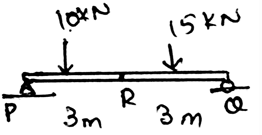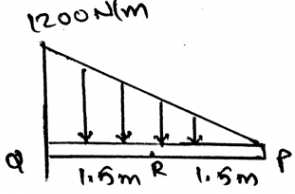Courses

# Test: Resultant Of A General Distributed Loading

## 15 Questions MCQ Test Engineering Mechanics | Test: Resultant Of A General Distributed Loading

Description
This mock test of Test: Resultant Of A General Distributed Loading for Mechanical Engineering helps you for every Mechanical Engineering entrance exam. This contains 15 Multiple Choice Questions for Mechanical Engineering Test: Resultant Of A General Distributed Loading (mcq) to study with solutions a complete question bank. The solved questions answers in this Test: Resultant Of A General Distributed Loading quiz give you a good mix of easy questions and tough questions. Mechanical Engineering students definitely take this Test: Resultant Of A General Distributed Loading exercise for a better result in the exam. You can find other Test: Resultant Of A General Distributed Loading extra questions, long questions & short questions for Mechanical Engineering on EduRev as well by searching above.
QUESTION: 1

### The determination of the internal loading in the different structures is usually done so as to___________

Solution:

The determination of the internal forces in the structure is done so as to design the structure as in the application purpose the structure will be subjected to many loads. This will help us to make the beam properly. And also this will ensure that the structure will not break after the loading is done on them. For this another method is the distribution load method.

QUESTION: 2

### Determine the moment generated at R.Solution:

The loading acting upward is considered to be positive. This is done as to decrease the time used for the calculations. If the method of sections is being applied at the complex sections for the determination of the unknown forces, much time is required for it. Thus the main motto is to make the calculations easy and make the less use of time.

QUESTION: 3

### Choose the correct one.

Solution:

The coplanar system means the consideration is with the 2D only. No 3D. Thus if there is any moment needed to be considered we need to take it along the point and not along the axis. But talking about the axis, there might be no axis in the 2D, as it is only in the 3D.

QUESTION: 4

The slope of the shear diagram of the different structure is equal to__________

Solution:

After the application of the force equation of equilibrium to the segment of the structure, we have the above result. This is done on the very small part of the structure. That is the minimal section of the beam is to be considered and then the application of the equilibrium equations are done so as to calculate the final result.

QUESTION: 5

Free body diagrams doesn’t play any role in making the calculations on the conditions of the resultants of the body.

Solution:

The free body diagrams does play an important role in the formation of the conditions of the resultants of the rigid body. As the net forces are zero, the fbd helps us to take the measure of the same. That is to see whether the summation is really zero or not.

QUESTION: 6

The simplification of the forces on the axis is done as __________

Solution:

A particular system of the rules is followed that is if the upward direction is taken as positive then the downward direction is taken as negative. This is same as done with the couple in the 2D. That is the forces can be easily simplified. If taken in the vector form then the task is even easier.

QUESTION: 7

Determine the shear force of the beam shown.Solution:

The loading acting upward is considered to be positive. This is done as to decrease the time used for the calculations. If the method of sections is being applied at the complex sections for the determination of the unknown forces, much time is required for it. Thus the main motto is to make the calculations easy and make the less use of time.

QUESTION: 8

The net forces of acting on the body needs to be zero. This is also applicable for the simply supported structures. This means that the support reaction are also counted in making the net force zero.

Solution:

The support reactions of the beam is also counted in the making of the forces zero. As far as the net force is concerned the support reaction does affect the conditions for the equilibrium of the body. Hence one needs to take care of the support reactions of the structures too.

QUESTION: 9

What does the Newton’s third law states?

Solution:

The requirement of the third law is important in the determination of the resultants of the body. Specially the rigid bodies. The rigid body particles are in the equilibrium and are thus facing the forces and to be in the equilibrium they also react and apply the opposite force and thus the third law of newton.

QUESTION: 10

The ___________ forces do not cause the rotation.

Solution:

The concurrent forces are the which are somewhere touching the axis of rotation. If any of the force is touching that axis, that force is not considered, or is insufficient to cause a rotation. If a force is concurrent then the perpendicular distance of the force from the line of axis is zero, thus no rotation. As we know rotation is caused by moment.

QUESTION: 11

Which of the following is correct?

Solution:

The application of the conditions of the equilibrium of the body is valid throughout. This means that the conditions are irrespective of the types of forces. The conditions are the basic rules that defines the equilibrium of the body and thus are applicable in any type of forces of the real axis.

QUESTION: 12

For the conditions of the equilibrium of the body, i.e. the rigid body only the external forces defines the equilibrium. And the support reactions only cancels out the rotation part of the body.

Solution:

The application of the support reaction forces does affect the conditions of the equilibrium of the body. Not only the external but the support reaction forces that are developed by the sake of external forces does develop a tending effect on the equilibrium of the body. Thus the support reaction forces also cancels the external forces.

QUESTION: 13

The simplification of the couple for getting the resultant is done on the basis of the ___________

Solution:

The couple is simplified by taking the direction of the rotation of the body as positive or negative. That is if the clockwise direction is positive then the anti-clockwise direction is taken as negative. If the couple is a vector it can be easily simplified by taking the components.

QUESTION: 14

If the supports are properly aligned then the reaction forces developed are adequate to support the body.

Solution:

This is the basic nature observed during the experiments on the beams an all the support system. The dimension doesn’t affect the equilibrium conditions. The main motto is to achieve the equilibrium. It is achieved by equating the net force equal to zero.

QUESTION: 15

The moment is the cross product of which two vectors?

Solution:

The cross product needs to take in the proper sequence. If not taken then the answer is just the opposite of the true answer. That’s why, the answer is not the Force and Radius vectors, but the Radius and Force vectors. Because the moment has its direction, as many of the cross products have, and thus precaution needs to be taken.